Test: Measurement Of Length - 1

# Test: Measurement Of Length - 1

Test Description

## 10 Questions MCQ Test Mathematics for Class 3: NCERT | Test: Measurement Of Length - 1

Test: Measurement Of Length - 1 for Class 3 2022 is part of Mathematics for Class 3: NCERT preparation. The Test: Measurement Of Length - 1 questions and answers have been prepared according to the Class 3 exam syllabus.The Test: Measurement Of Length - 1 MCQs are made for Class 3 2022 Exam. Find important definitions, questions, notes, meanings, examples, exercises, MCQs and online tests for Test: Measurement Of Length - 1 below.
Solutions of Test: Measurement Of Length - 1 questions in English are available as part of our Mathematics for Class 3: NCERT for Class 3 & Test: Measurement Of Length - 1 solutions in Hindi for Mathematics for Class 3: NCERT course. Download more important topics, notes, lectures and mock test series for Class 3 Exam by signing up for free. Attempt Test: Measurement Of Length - 1 | 10 questions in 20 minutes | Mock test for Class 3 preparation | Free important questions MCQ to study Mathematics for Class 3: NCERT for Class 3 Exam | Download free PDF with solutions
 1 Crore+ students have signed up on EduRev. Have you?
Test: Measurement Of Length - 1 - Question 1

### Billu want to measure the length of a cloth. Which measuring instrument among the following he should use to measure the cloth?

Detailed Solution for Test: Measurement Of Length - 1 - Question 1

Since, cloth is very long in length so it should be measured using metre rod. Therefore, the answer is option (c) metre rod.

Test: Measurement Of Length - 1 - Question 2

### Pintu want to measure the length of different things. Match the things given in List I with the measuring instrument given in List II to help Pintu find the length of the, items.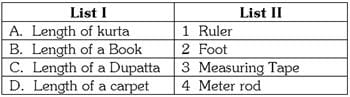Detailed Solution for Test: Measurement Of Length - 1 - Question 2

Since, length of a kurta is measured using measuring tape. So, A → 3.

Since, length of a book is measured using ruler. So, B → 1.

Since, length of a dupatta is measured using metre rod. So, C → 4.

Since, length of a carpet is measured using foot. So, D →  2.

Therefore, the answer is option (d) 3142

Test: Measurement Of Length - 1 - Question 3

### Donald duck is swimming in a pond from one place to another in the pond as given below. Can you find the distance he swim from the shore?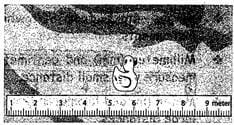Detailed Solution for Test: Measurement Of Length - 1 - Question 3

Since, the front of Donald duck ends at 6 cm on the scale so he swim at a distance of 6 cm from the shore. Therefore, the answer is option (c) 6 cm.

Test: Measurement Of Length - 1 - Question 4

Srishti bought a measuring tape from the market. She was amazed to see that some of the numbers were missing on the measuring tape. Can you find the numbers for her given by 'A' ?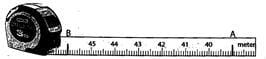Detailed Solution for Test: Measurement Of Length - 1 - Question 4

38, 39, 40, 41, 42, 43, 44, 45, 46

Test: Measurement Of Length - 1 - Question 5

Srishti bought a measuring tape from the market. She was amazed to see that some of the numbers were missing on the measuring tape. Can you find the numbers for her denoted by 'B'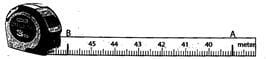Detailed Solution for Test: Measurement Of Length - 1 - Question 5

So, A →  39 B → 46

Test: Measurement Of Length - 1 - Question 6

Help Jiggy find the missing

Ruler : Centimeter : : Measuring Tape :?

Detailed Solution for Test: Measurement Of Length - 1 - Question 6

Since, Measuring tape measures in meter.
Therefore, the answer is option (b) Metre.

Test: Measurement Of Length - 1 - Question 7

Help Jiggy find the missing

Distance from one house to next house: Meters: : Distance from house to school : ?

Detailed Solution for Test: Measurement Of Length - 1 - Question 7

Since, distance from house to school is more, it will be in kilometers. Therefore, the answer is option (d) Kilometers

Test: Measurement Of Length - 1 - Question 8

Hathi bhai is a Carpenter. He wants to construct a door and a table. He has a Meter rod only to measure the length of wood needed. Can you tell which of the following will be more than 1 meter?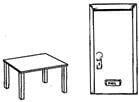Detailed Solution for Test: Measurement Of Length - 1 - Question 8

Since, the door is long hence length of the door will be more than 1 metre. Therefore, the answer is option (a) length of the door.

Test: Measurement Of Length - 1 - Question 9

Direction: Banku is making a list of things whose length will be in centimeters and a list whose length will be in meters. Find the odd one among them in the following questions:

Things which will be in centimeters

Detailed Solution for Test: Measurement Of Length - 1 - Question 9

Since, length of a kurta will be in centimeters. Therefore, the answer is option (d) length of kurta.

Test: Measurement Of Length - 1 - Question 10

Direction: Banku is making a list of things whose length will be in centimeters and a list whose length will be in meters. Find the odd one among them in the following questions:

Things which will be in meters.

Detailed Solution for Test: Measurement Of Length - 1 - Question 10

Since, height of a cat will be in meters. Therefore, the answer is option (c) Height of a cat.

## Mathematics for Class 3: NCERT

50 videos|84 docs|44 tests
 Use Code STAYHOME200 and get INR 200 additional OFF Use Coupon Code
Information about Test: Measurement Of Length - 1 Page
In this test you can find the Exam questions for Test: Measurement Of Length - 1 solved & explained in the simplest way possible. Besides giving Questions and answers for Test: Measurement Of Length - 1, EduRev gives you an ample number of Online tests for practice

## Mathematics for Class 3: NCERT

50 videos|84 docs|44 tests